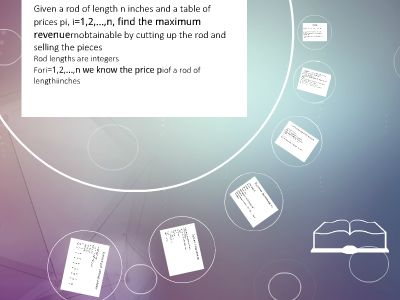Publications: 80 | Followers: 0

## Rod cutting - personal.utdallas.edu

Publish on Category: Birds 0

Rod cutting
Decide where to cut steel rods:Given a rod of length n inches and a table of prices pi,i=1,2,…,n, find the maximum revenuernobtainable by cutting up the rod and selling the piecesRod lengths are integersFori=1,2,…,n we know the price piof a rod of lengthiinches
Example
length I: 1 2 3 4 5 6 7 8 9 10------------------------------------------------price pi: 1 5 8 9 10 17 17 20 24 30For a rod of length 4: 2+2 is optimal (p2+p2=10)In general, can cut a rod of length n 2n-1ways
If optimal sol. cuts rod in k pieces thenoptimal decomposition: n=i1+i2+…+ikRevenue:rn=pi1+pi2+…+pikIn general:rn=max{pn,r1+rn-1,r2+rn-2,…,rn-1+r1}Initial cut of the rod: two pieces of sizeiand n-IRevenueriandrn-ifrom those two piecesNeed to consider all possible values ofiMay get better revenue if we sell the rod uncut
A different view of the problem
Decomposition inA first, left-hand piece of lengthiA right-hand reminder of length n-iOnly the reminder is further dividedThenrn=max{pi+rn-i, 1 <=i<= n}Thus, need solution to only onesubproblem
Top-down implementation
CUT-ROD(p,n)if n==0return 0q = -∞fori=1 to nq=max{q,p[i]+CUT-ROAD(p,n-i)}return qTime recurrence: T(n)=1+T(1)+T(2)+…+T(n-1)T(n)=O(2n)
Dynamic Programming
Optimality ofsubproblemsis obviousDP-CUT-ROD(p,n)let r[0..n], s[0..n] be new arraysr=0for j=1 to nq=-∞fori=1 to jif q < p[i]+r[j-i]s[j]=i;q= p[i]+r[j-i]r[j]=qreturn r and s
Retrieving an optimal solution
PRINT-CUT-ROD(r,s) = DP-CUT-ROD(p,n)while n>0print s[n]n=n-s[n]Example:i0 1 2 3 4 5 6 7r[i] 0 1 5 8 10 13 17 18s[i] 0 1 2 3 2 2 6 1

0

Embed

Share

Upload

Make amazing presentation for free
Rod cutting - personal.utdallas.edu